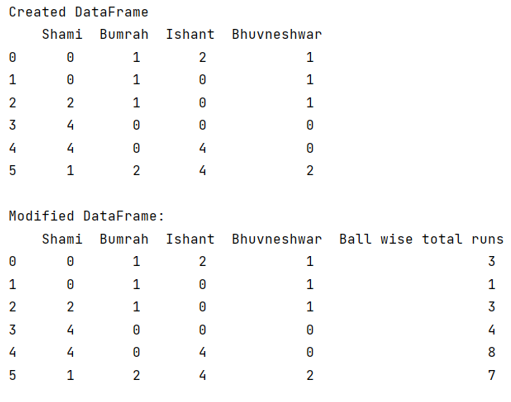# Pandas: Sum up multiple columns into one column without last column

Given a DataFrame, we need to create a new column in which contains sum of values of all the columns row wise. By Pranit Sharma Last updated : September 25, 2023

Pandas is a special tool that allows us to perform complex manipulations of data effectively and efficiently. Inside pandas, we mostly deal with a dataset in the form of DataFrame. DataFrames are 2-dimensional data structures in pandas. DataFrames consist of rows, columns, and data.

## Problem statement

Suppose, we have a dataframe that contains multiple columns of bowlers' names having their values containing runs on their six continue balls, we need to calculate the row-wise sum of all the balls except for the last column.

## Summing up multiple columns into one column without last column

For this purpose, we will use pandas.DataFrame.iloc property for slicing so that we can select from the first column to the second last column. Then we will use sum() method to calculate the sum and finally we will store all these values in a new column of the dataframe.

Note

To work with pandas, we need to import pandas package first, below is the syntax:

```import pandas as pd
```

Let us understand with the help of an example,

## Python program to create a new column in which contains sum of values of all the columns row-wise

```# Importing pandas package
import pandas as pd

# Creating a Dictionary
d = {
'Shami':[0,0,2,4,4,1],
'Bumrah':[1,1,1,0,0,2],
'Ishant':[2,0,0,0,4,4],
'Bhuvneshwar':[1,1,1,0,0,2]
}

# Creating a DataFrame
df = pd.DataFrame(d)

# Display DataFrame
print("Created DataFrame\n",df,"\n")

# Creating new column n which sum of all columns
# will be assigned except last column
df['Ball wise total runs']= df.iloc[:, -4:-1].sum(axis=1)

# Display modified dataframe
print("Modified DataFrame:\n",df)
```

### Output

The output of the above program is: